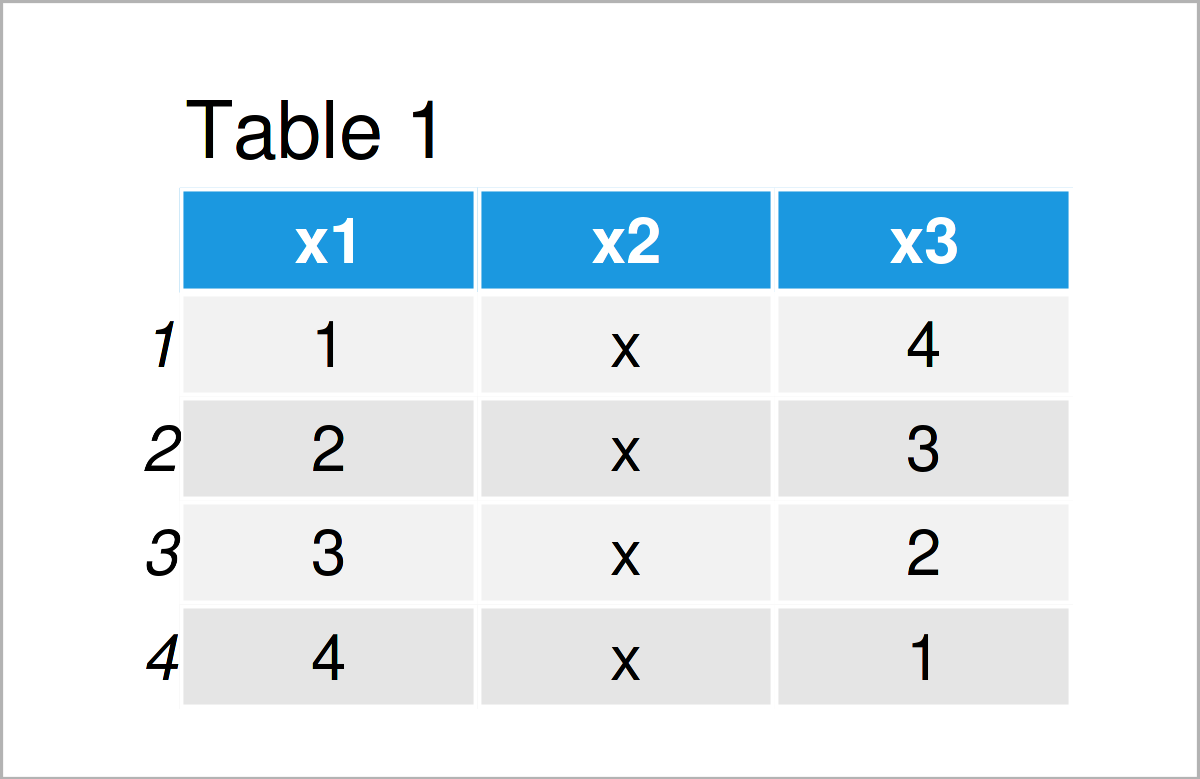# Delete Data Frame from Workspace in R (3 Examples)

In this tutorial, I’ll illustrate how to remove a data frame from the workspace in the R programming language.

The article contains these topics:

## Construction of Example Data

First of all, we’ll have to construct some example data:

```data_1 <- data.frame(x1 = 1:4,      # Create first data frame
x2 = "x",
x3 = 4:1)
data_1                              # Print first data frame``````data_2 <- data.frame(y1 = 10:14,    # Create second data frame
y2 = "y",
y3 = 14:10,
y4 = "yy")
data_2                              # Print second data frame``````data_3 <- data.frame(z1 = 1:6,      # Create third data frame
z2 = 7:12)
data_3                              # Print third data frame```As shown in Tables 1-3, we have created three different data frames called data_1, data_2, and data_3 by executing the previous R programming code.

Let’s also create some other types of data objects:

```vec1 <- 1:5                         # Create first vector object
vec1                                # Print first vector object
#  1 2 3 4 5```
```vec2 <- LETTERS[1:5]                # Create second vector object
vec2                                # Print second vector object
#  "A" "B" "C" "D" "E"```

The previous R code has created two vectors. So at this point of the tutorial, our workspace contains three data frames and two vectors.

Let’s remove some of these data!

## Example 1: Drop Single Data Frame from Workspace

In Example 1, I’ll show how to delete only one specific data frame from the global environment in R.

For this task, we can apply the rm function as shown below:

`rm(data_1)                          # Remove one data frame`

After executing the R syntax above, the data frame object data_1 is dropped from the workspace.

## Example 2: Drop Multiple Data Frames from Workspace

In Example 2, I’ll show how to delete multiple data frame objects.

Let’s first re-create the first data frame data_1 that we have removed in Example 1:

```data_1 <- data.frame(x1 = 1:4,      # Re-create first data frame
x2 = "x",
x3 = 4:1)```

Now, we can apply the rm function once again to clear two data frames from the workspace at the same time:

`rm("data_1", "data_3")              # Remove two data frames`

At this point, the data frames data_1 and data_3 have been removed.

## Example 3: Drop All Data Frames from Workspace

In this example, I’ll explain how to drop all data objects with the data.frame class simultaneously.

First, we have to re-create the two example data frame data_1 and data_3:

```data_1 <- data.frame(x1 = 1:4,      # Re-create first data frame
x2 = "x",
x3 = 4:1)```
```data_3 <- data.frame(z1 = 1:6,      # Re-create third data frame
z2 = 7:12)```

Now, we can use the rm, ls, sapply, mget, and class functions to delete all data frames from the workspace:

```rm(list = ls()[sapply(mget(ls()),   # Remove each data frame
class) == "data.frame"])```

After running the previous R code, only the two vector objects are kept in the workspace.

## Video & Further Resources

I have recently published a video on my YouTube channel, which shows the examples of this tutorial. Please find the video below.

Please accept YouTube cookies to play this video. By accepting you will be accessing content from YouTube, a service provided by an external third party.If you accept this notice, your choice will be saved and the page will refresh.

In addition, you might have a look at the related tutorials on www.statisticsglobe.com.

To summarize: In this tutorial, I have explained how to drop a data frame from the global environment in R. Please let me know in the comments section below, if you have additional questions.

Subscribe to the Statistics Globe Newsletter